Resources:

Have you found videos, websites, or explanations that helped you understand this chapter? Let us know and we'll add them to "Resources" part of this page for other students to use.

All answers and explanations are available !

Question 1

Arrange the following series of compounds in order of increasing lattice energies.
Select one:Explanation

Smaller the radius of element = higher lattice energy

smaller the radius the stronger the bond.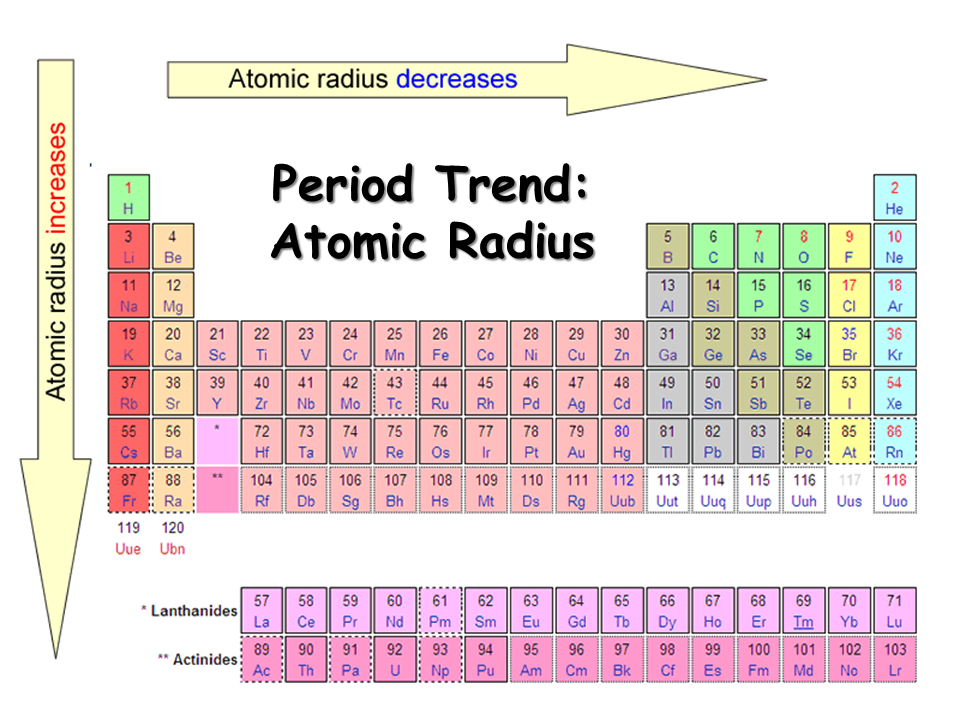Question 2

Arrange the following series of compounds in order of increasing lattice energies.
Select one:Explanation

Smaller the radius of element = higher lattice energy

smaller the radius the stronger the bond.Question 3

Arrange the following series of compounds in order of increasing lattice energies.
Select one:Explanation

Smaller the radius of element = higher lattice energy

smaller the radius the stronger the bond.Question 4

Write Lewis structures for the following compounds. Match compounds with the same Lewis Structure. All must be correct to get credit.

 LiF Answer 1 Choose...NaClFBrCOO2Cl2 Answer 2 Choose...NaClFBrCOO2NO+ Answer 3 Choose...NaClFBrCOO2NO- Answer 4 Choose...NaClFBrCOO2Explanation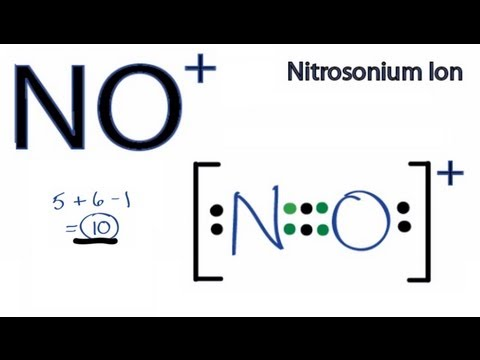Question 5

Write the Lewis structure for BF4- (each F is bonded to B). How many single bonds are there?Explanation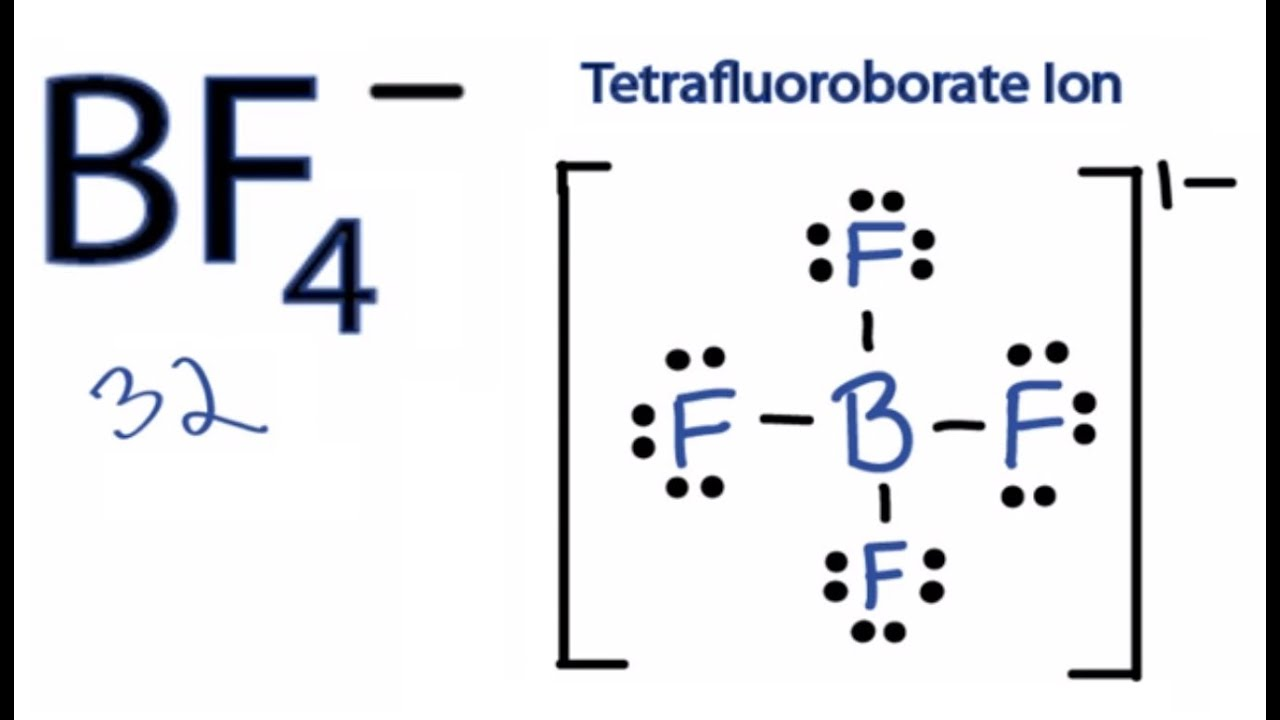Question 6

Write the Lewis structure for CH2CHCHO . How many single bonds are there?

Help--skeleton structure: CH2-CH-CHO, meaning the first C is bonded to 2 H's and a 2nd C. The 2nd C is also bonded to a H and the 3rd C. The 3rd C is also bonded to a H and an O.ExplanationQuestion 7

How many double bonds are there in CH2CHCHO?ExplanationQuestion 8

How many triple bonds are there in CH2CHCHO?ExplanationQuestion 9

Write the Lewis structure for CF2CF2 . How many single bonds are there?

Help--skeleton structure: CF2-CF2 meaning, The first C is bonded to two F's and the second C. In addition, the second C is bonded to two F's.ExplanationQuestion 10

Write the Lewis structure for CH2CHCCH How many single bonds are there?

Help--skeleton structure CH2-CH-C-CH.ExplanationQuestion 11

How many double bonds are there in CH2CHCCH?ExplanationQuestion 12

How many triple bonds are there in CH2CHCCH?ExplanationQuestion 13

Is the following Lewis structure correct for CH2CHOCOOH? Check all that apply.

Help given in feedback.Select one or more:Explanation

Formal charge = number of valence electrons -
(number of nonbonding electrons + number of bonding electrons/2)

C : 4 - 0 - 5 = -1

O: 6 - 4 -3 = -1

Question 14

Is the following Lewis structure correct for CH2CHOCOOH?Select one:Explanation

Formal charge = number of valence electrons -
(number of nonbonding electrons + number of bonding electrons/2)

Formal charge on elements are equal to zero similar to the formula.

Question 15

Is the following Lewis structure correct for CH2CHOCOOH? Check all that apply.Select one or more:Explanation

Formal charge = number of valence electrons -
(number of nonbonding electrons + number of bonding electrons/2)

O : 6 - 6 - 1 = -1

C: 4 - 3 = +1

Question 16

Is the following Lewis structure correct for CH2CHOCOOH? Check all that apply.Select one or more:Explanation

O : 6 - 6 - 1 = -1

Charge of Lewis structure (-1) not equal to formula's charge (0)

Question 17

Which of the following molecules has the most polar bond: O2, BrCl, or ICl?
Select one:Explanation

The greater the difference in electronegativity, the greater the bond polarity.Question 18

There are two equivalent resonance structures for CHOO- (all atoms bonded to C). How many valence electrons are there? Remember all C and O atoms obey the octet rule.Explanation

CHOO-  : 4 + 1 + 6 + 6 +1 = 18 valence electrons

Question 19

Describe the carbon-oxygen bonds in CHOO-.
Select one:Explanation

The resonance structures obey the octet rule (except H which has 2 electrons like He).Question 20

With respect to either resonnace structure, which of the atoms have a formal charge of 0 in CHOO-?

Select one or more:ExplanationQuestion 21

What is the sum of the formal charges on the H, C, and 2 O atoms in CHOO-?Explanation

The sum of charge is equal to the formula's overall charge which in this case is -1.

Question 22

How many valence electrons are in HONO (H-O-N-O).Explanation

H-O-N-O :  1 + 6 + 5 +  6 =  18 valence electrons

Question 23

Draw two resonance structures for HONO. In the more important structure, which of the atoms have a formal charge of 0? Remember all N and O atoms obey the octet rule.
Select one or more:ExplanationThe best structure is the one that corresponds to the overall charge of the compound.

Question 24

In the less important resonance structure for HONO, which of the atoms have a formal charge of 0?
Select one or more:ExplanationQuestion 25

How many valence electrons are there in ClO3OH (Cl is the central atom--each O is bonded to it. The H is bonded to one of the O's)?Explanation

ClO3OH :  7 + (3 x 6) + 6+1 = 42 valence electrons

Question 26

For ClO3OH, write a resonance structure with the octet rule satisfied for the central atom and a second resonance structure that minimizes formal charges. In the one that satisfies the octet rule, which atoms have a formal charge of zero?
Select one or more:Explanation---------------------O
----------------------l
-----------------O-Cl-O
----------------------l
---------------------OH

Question 27

In the one that satisfies the octet rule, what is the formal charge of the chlorine?Explanation

Look Above at question 26

Question 28

In the one that minimizes formal charges, which atoms have a formal charge of zero?
Select one or more:Explanation

By the way, the one that minimizes formal charge is expected to be the more important resonance structure.

Look Above at question 26

Question 29

In the one that minimizes the formal charge, how many double bonds does the chlorine atom make?Question 30

In the gas phase, the oxide N2O5 has a structure with an N-O-N core,and each N bonds to two additional O atoms. In contrast, in the solid phase the stable form is an ionic solid, (NO2+)(NO3- ). How many valence electrons does N2O5 have?ExplanationQuestion 31

Draw one of the Lewis structure of the N2O5. In each case one oxygen bridges the nitrogens (N-O-N single bond) and 2 other oxygens are bonded to each N. How many equivalent resonance structures are there that satisfy the octet rule and where O makes at most 2 bonds?Explanation

Move the double for each oxygen and nitrogen connection for resonance strucutureQuestion 32

How many double bonds does each N2O5 resonance structures have?ExplanationQuestion 33

Draw the dominant Lewis structure for NO2+. Note, that this ion is isoelectronic with CO2. How many double bonds does this resonance structures have?ExplanationQuestion 34

How many valence electrons does NO3- have?Explanation

NO3-  : 5+ (3 x 6) + 1 = 24 valence electrons

Question 35

How many equivalent resonance structures does NO3- have?ExplanationQuestion 36

The reaction of XeF6 + H2O → XeOF4 + HF.

Write Lewis dot structures for all the molecules.

In the case of Xe containing molecules, Xe is the central atom.

How many of the F atoms in XeF6 satisfy the octet rule and form one bond?

ExplanationQuestion 37

How many lone pairs of electrons does Xe have in XeF6?ExplanationQuestion 38

How many valence electrons does XeOF4?Explanation

XeOF4:  8 + 6 +  (4 x 7) = 42 valence electrons

Question 39

How many lone pairs of electrons does Xe have in XeOF4? (Use the structure where all the atoms have a formal charge of 0.)Explanation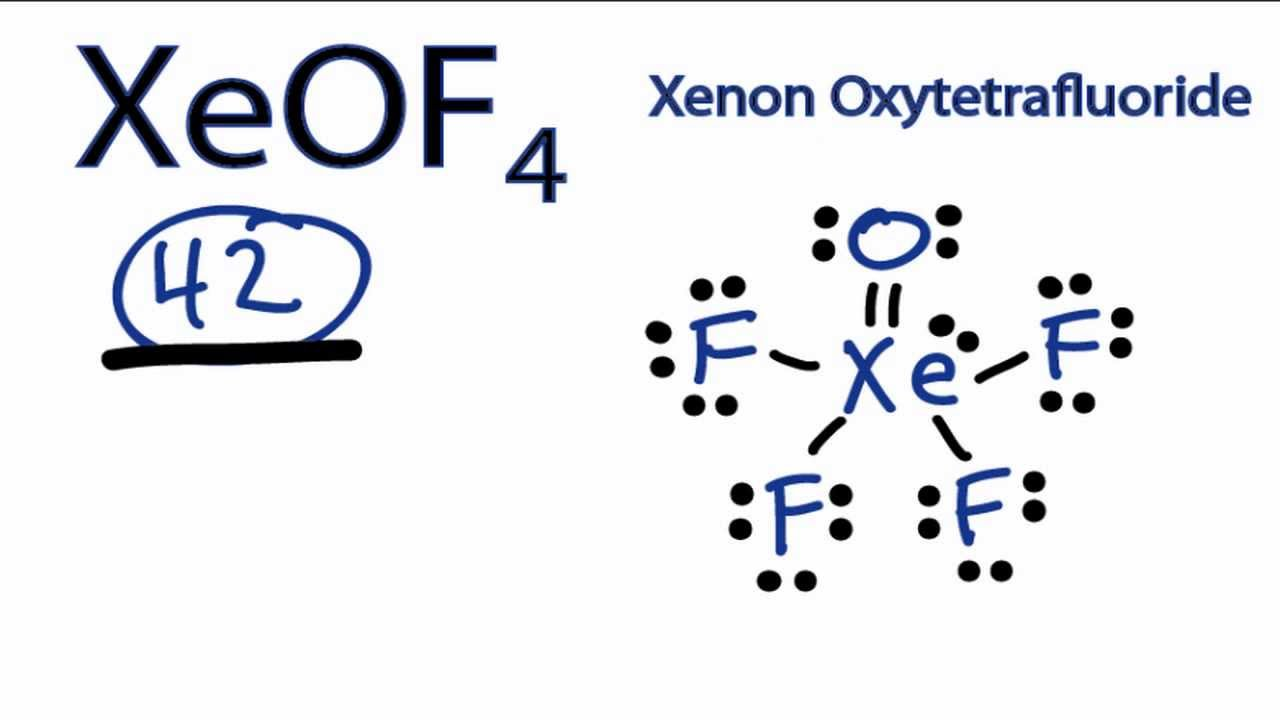Question 40

How many lone pairs of electrons does O have in XeOF4? (Use the structure where all the atoms have a formal charge of 0.)ExplanationQuestion 41

Using Table 9.4 (see below), calculate the energy (in kJ) required to break all of the bonds in 1 mole(s) of methanol (CH3OH).

Hint given in feedbackExplanationFirst calculate the per mole energy.

C- H : 414 kJ/mol   x 3  = 1242

C- O : 351 kJ/ mol   x 1 = 351

O - H : 463 kJ/ mol  x 1 = 463

------------------------------------------

2056 kJ/mol

Question 42

Using Table 9.4, calculate an approximate enthalpy (in kJ) for the reaction of 3.67 g gaseous methanol (CH3OH) in excess molecular oxygen to form gaseous carbon dioxide and gaseous water. (Hint, remember to first write the balanced equation.)Explanation

2CH3OH +3O2 --> 2CO2 +4H2O

sum of bonds reactants: 2*(3CH + CO + OH) + 3(O2)
Sum of bond products: 2*(2CO) + 4*(2OH)
2*(3*414 + 351 + 463) + (3*498) = 5606 kJ/mol reactant
4*799 + 8*463 =  6900 kJ /mol product

Now use the equation = rect - prod = -1294 kJ
-1294 kJ/ 2 mol CH3OH

-647 kJ / 1 mol  CH3OH

so no. of moles of methanol = 3.67/32 = 0.1147

So Enthalpy = -647 *0.1147 = -74.3

Question 43

Use Table 9.4 to calculate an approximate enthalpy or heat of reaction for the combustion of one mole of methane gas (CH4) to form gaseous H2O and CO2. What Volume (in L) of methane is needed to produce 3098 kJ of heat for methane gas at 31 oC and 7.89 atm pressure?

Hint given in feedbackExplanation

2) Balanced chemiacl reaction is

CH4 + 2O2  CO2 + 2H2O

H0 =  H0 (Reactant bonds) - H0 (product bonds)

H0 =. [4 H0(C - H) + 2H0(O = O) ] - [2 H0(C = O) + 4H0(O - H) ]

Substitute the bond enthalpy in above equation

[4(414 kJ/mol) + 2(498 KJ/mpl)] - [2(799 kJ/mol) + 4( 463 kJ/mol) ]

(2652 kJ/mol) - (3450) = -798 kJ/mol

H0 for your given reaction is = -798 kJ/mol

1 mole methane produce -798 kJ heat then to produce 3098 kJ heat required methane = 3098  / 798 = 3.88 mole of methane

volume fo methane in liter =

We know that PV = nRT

V = nRT/P

n = 3.88 mole,

T = 190C = 31+273.15 = 304 K,

P= 7.89 atm,

R = 0.08206 L atm mol-1 K-1 ( R = gas constant)

V = ?

Substitute these value in above equation.

V = 3.88 X 0.08205 X 304/7.89  = 12.27 L

12.27 L methane required

Question 44

What molecular compound is expected to be form from nitrogen and chlorine based on Lewis structures.. For NxCly, what is x+y?Explanation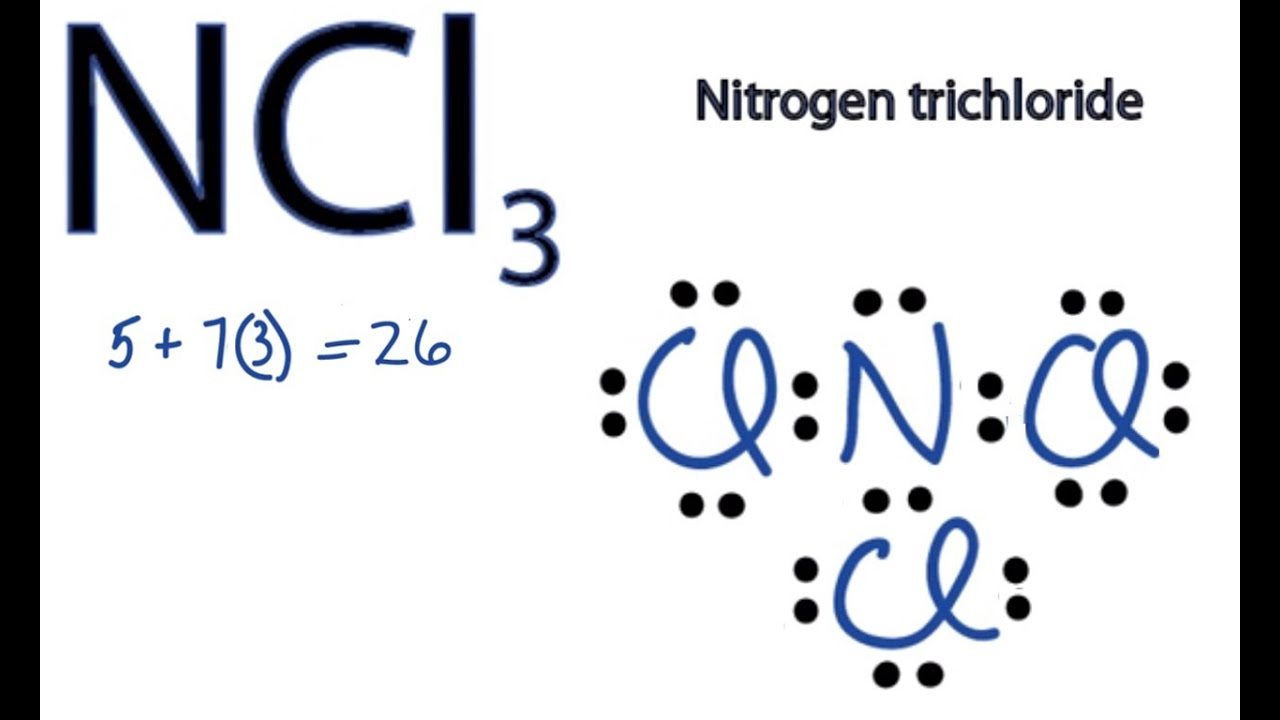NCl3: 1 + 3 = 4